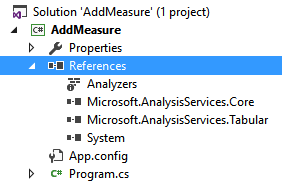I worked in several Analysis Services projects where certain metrics were developed outside of the regular database modeling development process. There are many examples for that:

• An add-in or an application that allows an end user to create a new DAX measure.
• A script that generates different versions of an existing measure to support common transformations (year-to-date, year-over-year, same-store-sales, …).

The new compatibility model (1200) in Analysis Services 2016 uses JSON as a format to store the metadata of a model. However, what is more important is that the object model for Tabular has now a one-to-one mapping between physical entities in the database and objects that you define in the model. This is not the same in the old compatibility model (110x) used by Analysis Services 2012/2014, where a certain object in the Tabular model (a table) corresponds to different physical entities in the object model used by Analysis Services (a dimension and a measure group). In order to simplify the access to older compatibility model (110x), you can use Tabular AMO 2012, a library available on CodePlex that exposes an object model that is close to the one used in the new version.

The easiest way to manipulate the new compatibility model programmatically is by using the entities of the Tabular Object Model (TOM), which is exposed as a new namespace in the latest Analysis Services Management Objects (AMO) library.

An alternative approach could be the one of using the Tabular Model Scripting Language (TMSL), but as you will see later this approach is not convenient in the current implementation.

At the end of this article you can download the sample file including the C# and PowerShell examples described in the next sections.

## Adding a new measure in C#

The class library including the TOM classes is included in the assembly Microsoft.AnalysisServices.Tabular, but you have to include also the Microsoft.AnalysisServices.Core assembly to connect to Analysis Services. Thus, in a new C# project, you have to include these two references, as you see in the following screenshot.In order to create a new measure, you have to connect to a server and create a new Measure object in the Measures collection of the table that will include such a definition. Even if the measure name has to be unique in the data model, a measure is created within a table, this also defines some semantic for Q&A in Power BI and for the drillthrough in Excel. Thus, you connect to the server, locate the database, the model, and the table. Then, you add the new measure in the Measures collection of the table. The following is an example of the code you can write.

```public static void AddMeasure() {
string serverName = @"localhost\tabular";
string databaseName = "Contoso";
string tableName = "Sales";
string measureName = "Total Sales";
string measureExpression = "SUMX ( Sales, Sales[Quantity] * Sales[Net Price] )";

string serverConnectionString = string.Format("Provider=MSOLAP;Data Source={0}", serverName);

Server server = new Server();
server.Connect(serverConnectionString);

Database db = server.Databases[databaseName];
Model model = db.Model;
Table table = model.Tables[tableName];
table.Measures.Add(new Measure() { Name = measureName, Expression = measureExpression });
model.SaveChanges();
}
```

If you want to modify an existing measure, you have to retrieve the measure object from the Measures collection using the method Find. The following code replaces an existing measure or creates a new one with the provided name in case it does not exist.

```public static void AddOrChangeMeasure() {
string serverName = @" localhost\tabular";
string databaseName = "Contoso";
string tableName = "Sales";
string measureName = "Total Sales";
string measureExpression = "SUMX ( Sales, Sales[Quantity] * Sales[Net Price] )";

string serverConnectionString = string.Format("Provider=MSOLAP;Data Source={0}", serverName);

Server server = new Server();
server.Connect(serverConnectionString);

Database db = server.Databases[databaseName];
Model model = db.Model;
Table table = model.Tables[tableName];
Measure measure = table.Measures.Find(measureName);
if (measure == null) {
measure = new Measure() { Name = measureName };
}
measure.Expression = measureExpression;
model.SaveChanges();
}
```

In both cases, it is important to call the method SaveChanges of the model object, because otherwise the change happens only in the client side version of the object model, but this is not transmitted to the server. Multiple changes are applied within the same transaction when you invoke SaveChanges.

## Adding a new measure in PowerShell

In PowerShell you have to load the assembly Microsoft.AnalysisServices.Tabular in order to access the TOM object.

In order to create a new measure, you use the same technique described for the C# code in the previous section. The following is an example of a PowerShell script that creates a new measure.

```[System.Reflection.Assembly]::LoadWithPartialName("Microsoft.AnalysisServices.Tabular")
\$serverName = ".\tab16";
\$databaseName = "Contoso";
\$tableName = "Sales";
\$measureName = "Total Sales";
\$measureExpression = "SUMX ( Sales, Sales[Quantity] * Sales[Net Price] )";

\$svr = new-Object Microsoft.AnalysisServices.Tabular.Server
\$svr.Connect(\$serverName)
\$database = \$svr.databases
\$db = \$database.GetByName(\$databaseName)
\$table = \$db.Model.Tables.Item(\$tableName)
\$measure = new-Object Microsoft.AnalysisServices.Tabular.Measure
\$measure.Name = \$measureName
\$measure.Expression = \$measureExpression
\$db.Model.SaveChanges()

```

Similar to what you have seen for C#, we have a PowerShell version of the script that replaces an existing measure or creates a new one if it does not exist.

```[System.Reflection.Assembly]::LoadWithPartialName("Microsoft.AnalysisServices.Tabular")
\$serverName = "localhost\tabular";
\$databaseName = "Contoso";
\$tableName = "Sales";
\$measureName = "Total Sales";
\$measureExpression = "SUMX ( Sales, Sales[Quantity] * Sales[Net Price] )";

\$svr = new-Object Microsoft.AnalysisServices.Tabular.Server
\$svr.Connect(\$serverName)
\$database = \$svr.databases
\$db = \$database.GetByName(\$databaseName)
\$table = \$db.Model.Tables.Item(\$tableName)
\$measure = \$table.Measures.Find(\$measureName)
if (\$measure -eq \$null) {
\$measure = new-Object Microsoft.AnalysisServices.Tabular.Measure
\$measure.Name = \$measureName